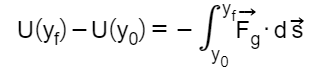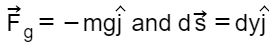# Problem: Consider a uniform gravitational field (a fair approximation near the surface of a planet). Find, whereExpress your answer in terms of m, g, y0, and yf.

###### FREE Expert Solution

$\begin{array}{rcl}{\stackrel{\mathbf{⇀}}{\mathbf{F}}}_{\mathbf{g}}\mathbf{·}{\mathbf{d}}_{\mathbf{s}}& \mathbf{=}& \mathbf{-}\mathbf{m}\mathbf{g}\stackrel{\mathbf{^}}{\mathbf{j}}\mathbf{·}\mathbf{d}\mathbf{y}\stackrel{\mathbf{^}}{\mathbf{j}}\\ & \mathbf{=}& \mathbf{-}\mathbf{m}\mathbf{g}\mathbf{d}\mathbf{y}\end{array}$###### Problem Details

Consider a uniform gravitational field (a fair approximation near the surface of a planet). Find, where